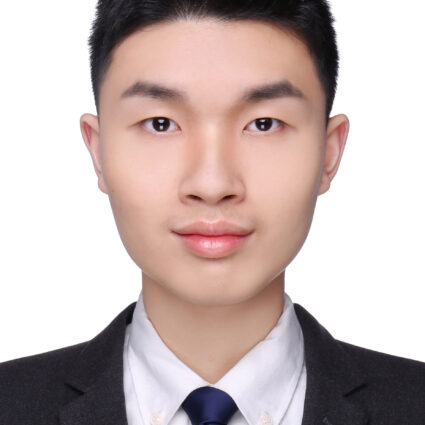# Xiang Li

Project Title: Quantum field theory of arithmetic moduli spaces

The number theory is at the core of the mathematics. And arithmetic geometry is a prevailing area of number theory with the powerful tool of algebraic geometry. The relation of geometry and number theory can be seen in a classic example, Mordell’s conjecture, relating the rational points with the genus. Surprisingly, besides geometry, quantum field theory which is originated from physics can be also involved in the research of number theory. Roughly speaking, this project will apply various ideas of quantum field theory to the study of moduli spaces arising in arithmetic geometry. To be more technical, the project will use spaces of Galois representations and spaces of principals bundles for sheaves of groups. A specific project will be to construct deformation quantisations of l-adic Poisson manifolds on which a cyclotomic Galois group will act as conformal symplectic automorphisms. The quantisation will also have an ‘equivariant’ nature, whereby the formal Planck constant will be scaled under this action. After suitable specialisation, it is hoped that the deformed algebra will admit a canonical representation with Z_p-action, using which one can construct new arithmetic invariants.Awarded:

Field:

University: